# Tests of Independence

#### 2022-04-21

OK, now we’re ready to do some analyses. This vignette focuses on relatively simple non-parametric tests and measures of association.

## CrossTable

For tabular displays, the CrossTable() function in the gmodels package produces cross-tabulations modeled after PROC FREQ in SAS or CROSSTABS in SPSS. It has a wealth of options for the quantities that can be shown in each cell.

Recall the GSS data used earlier.

# Agresti (2002), table 3.11, p. 106
GSS <- data.frame(
expand.grid(sex = c("female", "male"),
party = c("dem", "indep", "rep")),
count = c(279,165,73,47,225,191))

(GSStab <- xtabs(count ~ sex + party, data=GSS))
##         party
## sex      dem indep rep
##   female 279    73 225
##   male   165    47 191

Generate a crosstable showing cell frequency and the cell contribution to $$\chi^2$$.

# 2-Way Cross Tabulation
library(gmodels)
CrossTable(GSStab, prop.t=FALSE, prop.r=FALSE, prop.c=FALSE)
##
##
##    Cell Contents
## |-------------------------|
## |                       N |
## | Chi-square contribution |
## |-------------------------|
##
##
## Total Observations in Table:  980
##
##
##              | party
##          sex |       dem |     indep |       rep | Row Total |
## -------------|-----------|-----------|-----------|-----------|
##       female |       279 |        73 |       225 |       577 |
##              |     1.183 |     0.078 |     1.622 |           |
## -------------|-----------|-----------|-----------|-----------|
##         male |       165 |        47 |       191 |       403 |
##              |     1.693 |     0.112 |     2.322 |           |
## -------------|-----------|-----------|-----------|-----------|
## Column Total |       444 |       120 |       416 |       980 |
## -------------|-----------|-----------|-----------|-----------|
##
## 

There are options to report percentages (row, column, cell), specify decimal places, produce Chi-square, Fisher, and McNemar tests of independence, report expected and residual values (pearson, standardized, adjusted standardized), include missing values as valid, annotate with row and column titles, and format as SAS or SPSS style output! See help(CrossTable) for details.

## Chi-square test

For 2-way tables you can use chisq.test() to test independence of the row and column variable. By default, the $$p$$-value is calculated from the asymptotic chi-squared distribution of the test statistic. Optionally, the $$p$$-value can be derived via Monte Carlo simulation.

(HairEye <- margin.table(HairEyeColor, c(1, 2)))
##        Eye
## Hair    Brown Blue Hazel Green
##   Black    68   20    15     5
##   Brown   119   84    54    29
##   Red      26   17    14    14
##   Blond     7   94    10    16

chisq.test(HairEye)
##
##  Pearson's Chi-squared test
##
## data:  HairEye
## X-squared = 138.29, df = 9, p-value < 2.2e-16

chisq.test(HairEye, simulate.p.value = TRUE)
##
##  Pearson's Chi-squared test with simulated p-value (based on 2000
##  replicates)
##
## data:  HairEye
## X-squared = 138.29, df = NA, p-value = 0.0004998

## Fisher Exact Test

fisher.test(X) provides an exact test of independence. X must be a two-way contingency table in table form. Another form, fisher.test(X, Y) takes two categorical vectors of the same length.
For tables larger than $$2 \times 2$$ the method can be computationally intensive (or can fail) if the frequencies are not small.

fisher.test(GSStab)
##
##  Fisher's Exact Test for Count Data
##
## data:  GSStab
## p-value = 0.03115
## alternative hypothesis: two.sided

Fisher’s test is meant for tables with small total sample size. It generates an error for the HairEye data with $$n$$=592 total frequency.

fisher.test(HairEye)
## Error in fisher.test(HairEye): FEXACT error 6 (f5xact).  LDKEY=618 is too small for this problem: kval=238045028.
## Try increasing the size of the workspace.

## Mantel-Haenszel test and conditional association

Use the mantelhaen.test(X) function to perform a Cochran-Mantel-Haenszel $$\chi^2$$ chi test of the null hypothesis that two nominal variables are conditionally independent, $$A \perp B \; | \; C$$, in each stratum, assuming that there is no three-way interaction. X is a 3 dimensional contingency table, where the last dimension refers to the strata.

The UCBAdmissions serves as an example of a $$2 \times 2 \times 6$$ table, with Dept as the stratifying variable.

# UC Berkeley Student Admissions
##
##  Mantel-Haenszel chi-squared test with continuity correction
##
## Mantel-Haenszel X-squared = 1.4269, df = 1, p-value = 0.2323
## alternative hypothesis: true common odds ratio is not equal to 1
## 95 percent confidence interval:
##  0.7719074 1.0603298
## sample estimates:
## common odds ratio
##         0.9046968

The results show no evidence for association between admission and gender when adjusted for department. However, we can easily see that the assumption of equal association across the strata (no 3-way association) is probably violated. For $$2 \times 2 \times k$$ tables, this can be examined from the odds ratios for each $$2 \times 2$$ table (oddsratio()), and tested by using woolf_test() in vcd.

oddsratio(UCBAdmissions, log=FALSE)
##  odds ratios for Admit and Gender by Dept
##
##         A         B         C         D         E         F
## 0.3492120 0.8025007 1.1330596 0.9212838 1.2216312 0.8278727

lor <- oddsratio(UCBAdmissions)  # capture log odds ratios
summary(lor)
##
## z test of coefficients:
##
##    Estimate Std. Error z value  Pr(>|z|)
## A -1.052076   0.262708 -4.0047 6.209e-05 ***
## B -0.220023   0.437593 -0.5028    0.6151
## C  0.124922   0.143942  0.8679    0.3855
## D -0.081987   0.150208 -0.5458    0.5852
## E  0.200187   0.200243  0.9997    0.3174
## F -0.188896   0.305164 -0.6190    0.5359
## ---
## Signif. codes:  0 '***' 0.001 '**' 0.01 '*' 0.05 '.' 0.1 ' ' 1

##
##  Woolf-test on Homogeneity of Odds Ratios (no 3-Way assoc.)
##
## X-squared = 17.902, df = 5, p-value = 0.003072

## Some plot methods

### Fourfold displays

We can visualize the odds ratios of Admission for each department with fourfold displays using fourfold(). The cell frequencies $$n_{ij}$$ of each $$2 \times 2$$ table are shown as a quarter circle whose radius is proportional to $$\sqrt{n_{ij}}$$, so that its area is proportional to the cell frequency.

UCB <- aperm(UCBAdmissions, c(2, 1, 3))
dimnames(UCB)[] <- c("Yes", "No")
names(dimnames(UCB)) <- c("Sex", "Admit?", "Department")

Confidence rings for the odds ratio allow a visual test of the null of no association; the rings for adjacent quadrants overlap iff the observed counts are consistent with the null hypothesis. In the extended version (the default), brighter colors are used where the odds ratio is significantly different from 1. The following lines produce .

col <- c("#99CCFF", "#6699CC", "#F9AFAF", "#6666A0", "#FF0000", "#000080")
fourfold(UCB, mfrow=c(2,3), color=col)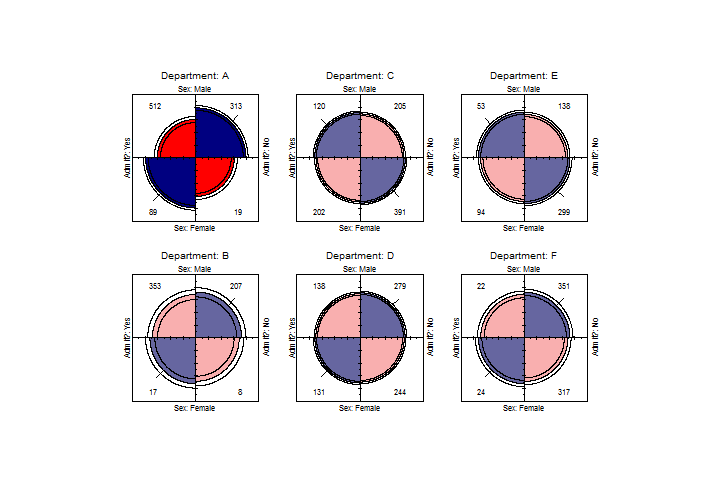Fourfold display for the UCBAdmissions data. Where the odds ratio differs significantly from 1.0, the confidence bands do not overlap, and the circle quadrants are shaded more intensely.

Another vcd function, cotabplot(), provides a more general approach to visualizing conditional associations in contingency tables, similar to trellis-like plots produced by coplot() and lattice graphics. The panel argument supplies a function used to render each conditional subtable. The following gives a display (not shown) similar to .

cotabplot(UCB, panel = cotab_fourfold)

### Doubledecker plots

When we want to view the conditional probabilities of a response variable (e.g., Admit) in relation to several factors, an alternative visualization is a doubledecker() plot. This plot is a specialized version of a mosaic plot, which highlights the levels of a response variable (plotted vertically) in relation to the factors (shown horizontally). The following call produces , where we use indexing on the first factor (Admit) to make Admitted the highlighted level.

In this plot, the association between Admit and Gender is shown where the heights of the highlighted conditional probabilities do not align. The excess of females admitted in Dept A stands out here.

doubledecker(Admit ~ Dept + Gender, data=UCBAdmissions[2:1,,])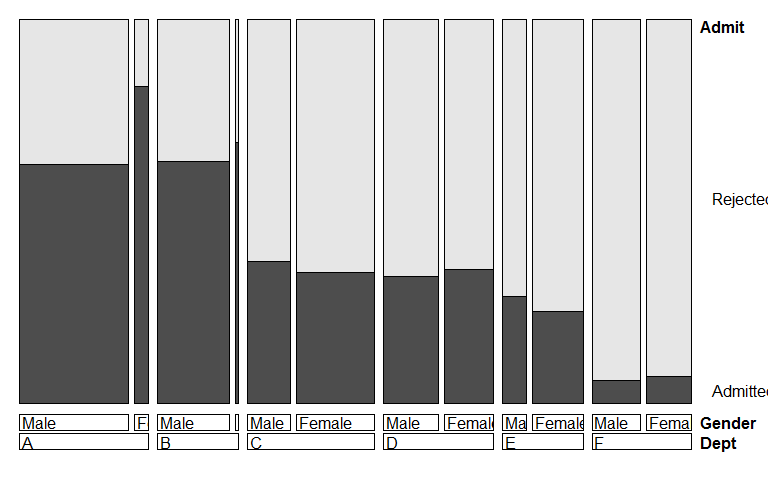Doubledecker display for the UCBAdmissions data. The heights of the highlighted bars show the conditional probabilities of Admit, given Dept and Gender.

### Odds ratio plots

Finally, the there is a plot() method for oddsratio objects. By default, it shows the 95% confidence interval for the log odds ratio. is produced by:

plot(lor,
xlab="Department",
ylab="Log Odds Ratio (Admit | Gender)")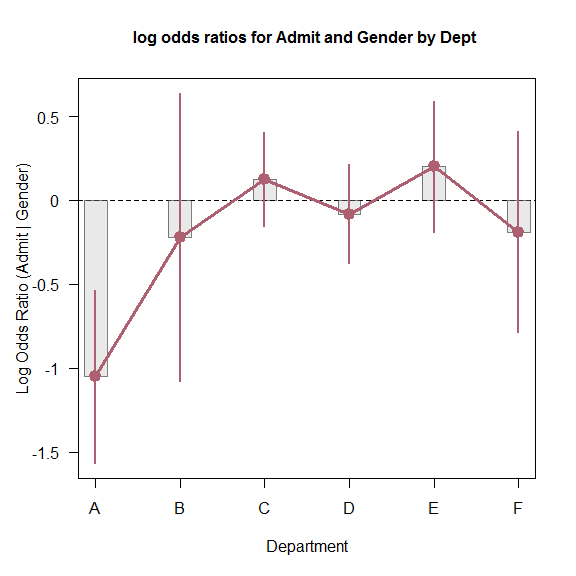Log odds ratio plot for the UCBAdmissions data.

{#fig:oddsratio}

## Cochran-Mantel-Haenszel tests for ordinal factors

The standard $$\chi^2$$ tests for association in a two-way table treat both table factors as nominal (unordered) categories. When one or both factors of a two-way table are quantitative or ordinal, more powerful tests of association may be obtaianed by taking ordinality into account, using row and or column scores to test for linear trends or differences in row or column means.

More general versions of the CMH tests (Landis etal., 1978) (Landis, Heyman, and Koch 1978) are provided by assigning numeric scores to the row and/or column variables. For example, with two ordinal factors (assumed to be equally spaced), assigning integer scores, 1:R and 1:C tests the linear $$\times$$ linear component of association. This is statistically equivalent to the Pearson correlation between the integer-scored table variables, with $$\chi^2 = (n-1) r^2$$, with only 1 $$df$$ rather than $$(R-1)\times(C-1)$$ for the test of general association.

When only one table variable is ordinal, these general CMH tests are analogous to an ANOVA, testing whether the row mean scores or column mean scores are equal, again consuming fewer $$df$$ than the test of general association.

The CMHtest() function in vcdExtra calculates these various CMH tests for two possibly ordered factors, optionally stratified other factor(s).

Example:

Recall the $$4 \times 4$$ table, JobSat introduced in @(sec:creating),

JobSat
##         satisfaction
## income   VeryD LittleD ModerateS VeryS
##   < 15k      1       3        10     6
##   15-25k     2       3        10     7
##   25-40k     1       6        14    12
##   > 40k      0       1         9    11

Treating the satisfaction levels as equally spaced, but using midpoints of the income categories as row scores gives the following results:

CMHtest(JobSat, rscores=c(7.5,20,32.5,60))
## Cochran-Mantel-Haenszel Statistics for income by satisfaction
##
##                  AltHypothesis  Chisq Df     Prob
## cor        Nonzero correlation 3.8075  1 0.051025
## rmeans  Row mean scores differ 4.4774  3 0.214318
## cmeans  Col mean scores differ 3.8404  3 0.279218
## general    General association 5.9034  9 0.749549

Note that with the relatively small cell frequencies, the test for general give no evidence for association. However, the the cor test for linear x linear association on 1 df is nearly significant. The coin package contains the functions cmh_test() and lbl_test() for CMH tests of general association and linear x linear association respectively.

## Measures of Association

There are a variety of statistical measures of strength of association for contingency tables— similar in spirit to $$r$$ or $$r^2$$ for continuous variables. With a large sample size, even a small degree of association can show a significant $$\chi^2$$, as in the example below for the GSS data.

The assocstats() function in vcd calculates the $$\phi$$ contingency coefficient, and Cramer’s V for an $$r \times c$$ table. The input must be in table form, a two-way $$r \times c$$ table. It won’t work with GSS in frequency form, but by now you should know how to convert.

assocstats(GSStab)
##                     X^2 df P(> X^2)
## Likelihood Ratio 7.0026  2 0.030158
## Pearson          7.0095  2 0.030054
##
## Phi-Coefficient   : NA
## Contingency Coeff.: 0.084
## Cramer's V        : 0.085

For tables with ordinal variables, like JobSat, some people prefer the Goodman-Kruskal $$\gamma$$ statistic (Agresti 2002, 2.4.3) based on a comparison of concordant and discordant pairs of observations in the case-form equivalent of a two-way table.

GKgamma(JobSat)
## gamma        : 0.221
## std. error   : 0.117
## CI           : -0.009 0.451

A web article by Richard Darlington, [http://node101.psych.cornell.edu/Darlington/crosstab/TABLE0.HTM] gives further description of these and other measures of association.

## Measures of Agreement

The Kappa() function in the vcd package calculates Cohen’s $$\kappa$$ and weighted $$\kappa$$ for a square two-way table with the same row and column categories (Cohen 1960). Normal-theory $$z$$-tests are obtained by dividing $$\kappa$$ by its asymptotic standard error (ASE). A confint() method for Kappa objects provides confidence intervals.

data(SexualFun, package = "vcd")
(K <- Kappa(SexualFun))
##             value     ASE     z Pr(>|z|)
## Unweighted 0.1293 0.06860 1.885 0.059387
## Weighted   0.2374 0.07832 3.031 0.002437
confint(K)
##
## Kappa                 lwr       upr
##   Unweighted -0.005120399 0.2637809
##   Weighted    0.083883432 0.3908778

A visualization of agreement (Bangdiwala 1987), both unweighted and weighted for degree of departure from exact agreement is provided by the agreementplot() function. shows the agreementplot for the SexualFun data, produced as shown below.

The Bangdiwala measures (returned by the function) represent the proportion of the shaded areas of the diagonal rectangles, using weights $$w_1$$ for exact agreement, and $$w_2$$ for partial agreement one step from the main diagonal.

agree <- agreementplot(SexualFun, main="Is sex fun?")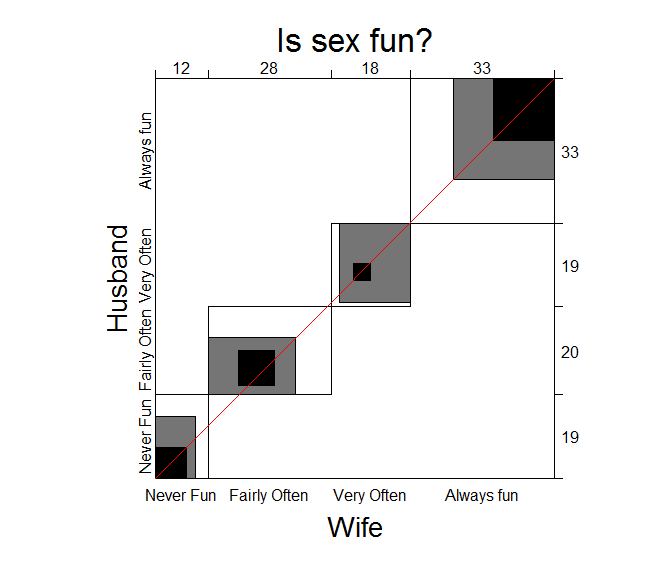Agreement plot for the SexualFun data.

unlist(agree)
##          Bangdiwala Bangdiwala_Weighted            weights1            weights2
##           0.1464624           0.4981723           1.0000000           0.8888889

In other examples, the agreement plot can help to show sources of disagreement. For example, when the shaded boxes are above or below the diagonal (red) line, a lack of exact agreement can be attributed in part to different frequency of use of categories by the two raters– lack of marginal homogeneity.

## Correspondence analysis

Correspondence analysis is a technique for visually exploring relationships between rows and columns in contingency tables. The ca package gives one implmentation. For an $$r \times c$$ table, the method provides a breakdown of the Pearson $$\chi^2$$ for association in up to $$M = \min(r-1, c-1)$$ dimensions, and finds scores for the row ($$x_{im}$$) and column ($$y_{jm}$$) categories such that the observations have the maximum possible correlations.%1

Here, we carry out a simple correspondence analysis of the HairEye data. The printed results show that nearly 99% of the association between hair color and eye color can be accounted for in 2 dimensions, of which the first dimension accounts for 90%.

library(ca)
ca(HairEye)
##
##  Principal inertias (eigenvalues):
##            1        2        3
## Value      0.208773 0.022227 0.002598
## Percentage 89.37%   9.52%    1.11%
##
##
##  Rows:
##             Black     Brown       Red    Blond
## Mass     0.182432  0.483108  0.119932 0.214527
## ChiDist  0.551192  0.159461  0.354770 0.838397
## Inertia  0.055425  0.012284  0.015095 0.150793
## Dim. 1  -1.104277 -0.324463 -0.283473 1.828229
## Dim. 2   1.440917 -0.219111 -2.144015 0.466706
##
##
##  Columns:
##             Brown     Blue     Hazel     Green
## Mass     0.371622 0.363176  0.157095  0.108108
## ChiDist  0.500487 0.553684  0.288654  0.385727
## Inertia  0.093086 0.111337  0.013089  0.016085
## Dim. 1  -1.077128 1.198061 -0.465286  0.354011
## Dim. 2   0.592420 0.556419 -1.122783 -2.274122

The resulting ca object can be plotted just by running the plot() method on the ca object, giving the result in . plot.ca() does not allow labels for dimensions; these can be added with title(). It can be seen that most of the association is accounted for by the ordering of both hair color and eye color along Dimension 1, a dark to light dimension.

plot(ca(HairEye), main="Hair Color and Eye Color")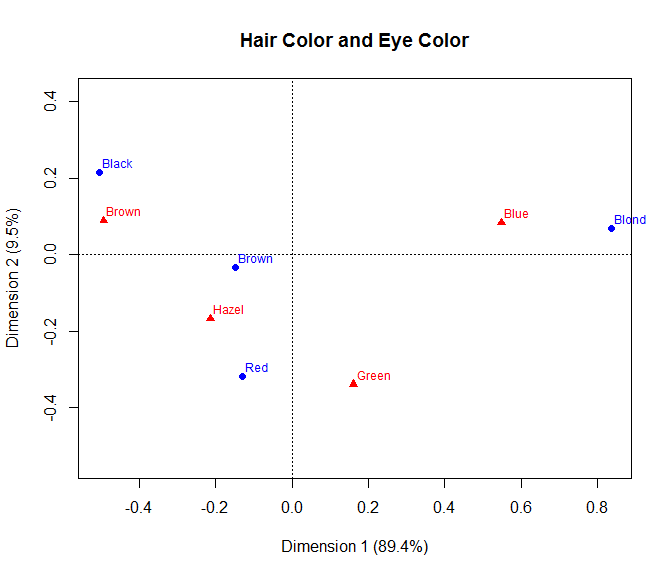Correspondence analysis plot for the HairEye data

1. Related methods are the non-parametric CMH tests using assumed row/column scores (), the analogous glm() model-based methods (), and the more general RC models which can be fit using gnm(). Correspondence analysis differs in that it is a primarily descriptive/exploratory method (no significance tests), but is directly tied to informative graphic displays of the row/column categories.↩︎